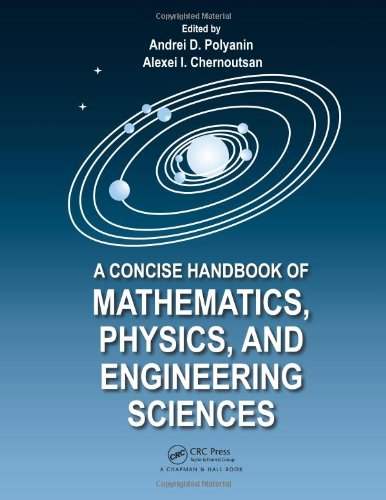# A Concise Handbook of Mathematics, Physics, and Engineering Sciences

• Length: 1125 pages
• Edition: 1
• Publisher:
• Publication Date: 2010-10-18
• ISBN-10: 143980639X
• ISBN-13: 9781439806395
Description

A Concise Handbook of Mathematics, Physics, and Engineering Sciences takes a practical approach to the basic notions, formulas, equations, problems, theorems, methods, and laws that most frequently occur in scientific and engineering applications and university education. The authors pay special attention to issues that many engineers and students find difficult to understand.

The first part of the book contains chapters on arithmetic, elementary and analytic geometry, algebra, differential and integral calculus, functions of complex variables, integral transforms, ordinary and partial differential equations, special functions, and probability theory. The second part discusses molecular physics and thermodynamics, electricity and magnetism, oscillations and waves, optics, special relativity, quantum mechanics, atomic and nuclear physics, and elementary particles. The third part covers dimensional analysis and similarity, mechanics of point masses and rigid bodies, strength of materials, hydrodynamics, mass and heat transfer, electrical engineering, and methods for constructing empirical and engineering formulas.

The main text offers a concise, coherent survey of the most important definitions, formulas, equations, methods, theorems, and laws. Numerous examples throughout and references at the end of each chapter provide readers with a better understanding of the topics and methods. Additional issues of interest can be found in the remarks. For ease of reading, the supplement at the back of the book provides several long mathematical tables, including indefinite and definite integrals, direct and inverse integral transforms, and exact solutions of differential equations.

Part I: Mathematics
Chapter M1: Arithmetic and Elementary Algebra
Chapter M2: Elementary Functions
Chapter M3: Elementary Geometry
Chapter M4: Analytic Geometry
Chapter M5: Algebra
Chapter M6: Limits and Derivatives
Chapter M7: Integrals
Chapter M8: Series
Chapter M9: Functions of Complex Variable
Chapter M10: Integral Transforms
Chapter M11: Ordinary Differential Equations
Chapter M12: Partial Differential Equations
Chapter M13: Special Functions and Their Properties
Chapter M14: Probability Theory

Part II: Physics
Chapter P1: Physical Foundations of Mechanics
Chapter P2: Molecular Physics and Thermodynamics
Chapter P3: Electrodynamics
Chapter P4: Oscillations and Waves
Chapter P5: Optics
Chapter P6: Quantum Mechanics. Atomic Physics
Chapter P7: Quantum Theory of Crystals
Chapter P8: Elements of Nuclear Physics

Part III: Elements of Applied andEngineering Sciences
Chapter E1: Dimensions and Similarity
Chapter E2: Mechanics of Point Particles and Rigid Bodies
Chapter E3: Elements of Strength of Materials
Chapter E4: Hydrodynamics
Chapter E5: Mass and Heat Transfer
Chapter E6: Electrical Engineering
Chapter E7: Empirical and Engineering Formulasand Criteria for Their Applicability

Part IV: Supplements
Chapter S1: Integrals
Chapter S2: Integral Transforms
Chapter S3: Orthogonal CurvilinearSystems of Coordinates
Chapter S4: Ordinary Differential Equations
Chapter S5: Some Useful ElectronicMathematical Resources
Chapter S6: Physical Tables
Chapter S7: Periodic Table